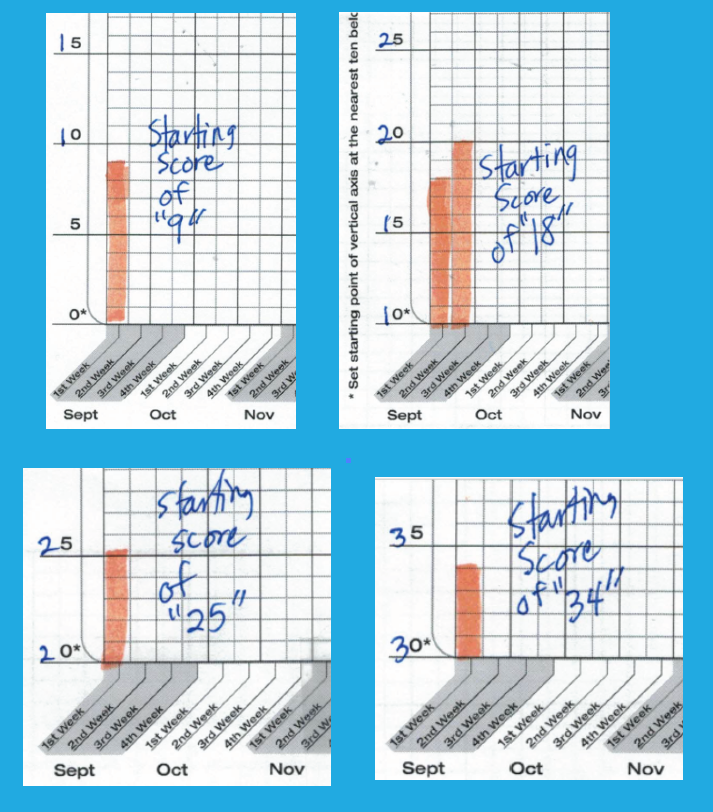## How do you complete the Individual Student graph?

Here are four examples of how to complete the vertical axis on the Individual Student Graph.

Amy writes:
I have a question about the Individual Student graph form. Can you send me example of a completed graph? I understand marking 10 points lower but the 0…5…..0…5….0…5 axis confused me.

Amy,
Here are some examples of how you would fill out the vertical axis of the Individual Student Graph depending on what the student’s starting score was on the Two-Minute Timings. The form says, “Set starting point of vertical axis at the nearest ten below the student’s first 2-minute timing (e.g., if first timing is 37, begin graph at 30, etc.).”

If a picture is worth a thousand words, then these four examples should make the procedure clearer. Thanks for asking for examples–which is often the best way to explain/teach something!

If the starting score was 9, you would set the starting point of the vertical axis at zero.

If the starting score of the two-minute timing was 18, you would set the starting point of the vertical axis at ten.

If the starting score of the two-minute timing was 25 you would set the starting point of the vertical axis at twenty.

If the starting score of the two-minute timing was 34 you would set the starting point of the vertical axis at thirty.

## 2 thoughts on “How do you complete the Individual Student graph?”

1.Kim Neptune says:

I tried to print the student math graph but the link appears to be broken.
Question- do you have a digital version of the math graph to track data- that you can send me or I can download?